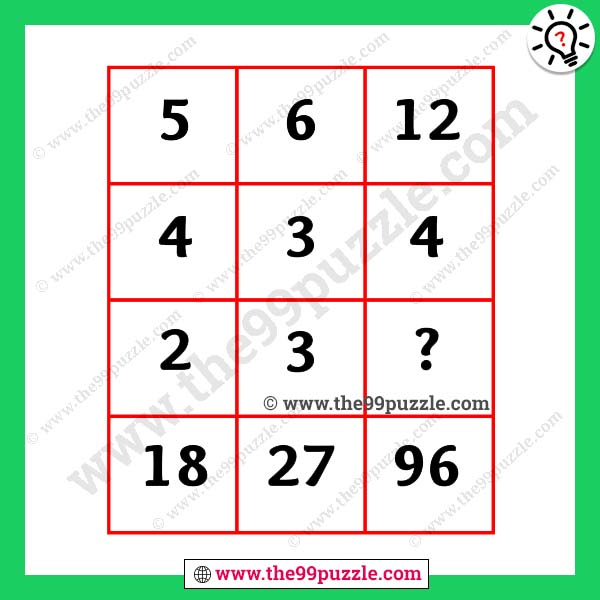# Brain stumping maths with answer-Puzz262

This math puzzle is fun and tricky. Puzzles lover wants to accept this math challenge. Many math teachers fail to answer this type of reasoning question. In this missing number puzzle, you see some numbers in the boxes. If you are a genius in mathematics you can easily find out the math logic and answer the brain stumping maths. These math puzzles question improves logical thinking. Think logically and mathematically to replace the question mark.###### Explanation:

1st Column = (5+4)×2 = 18

2nd Column = (6+3)×3 = 27

3rd Column = (12+4)×6 = 96